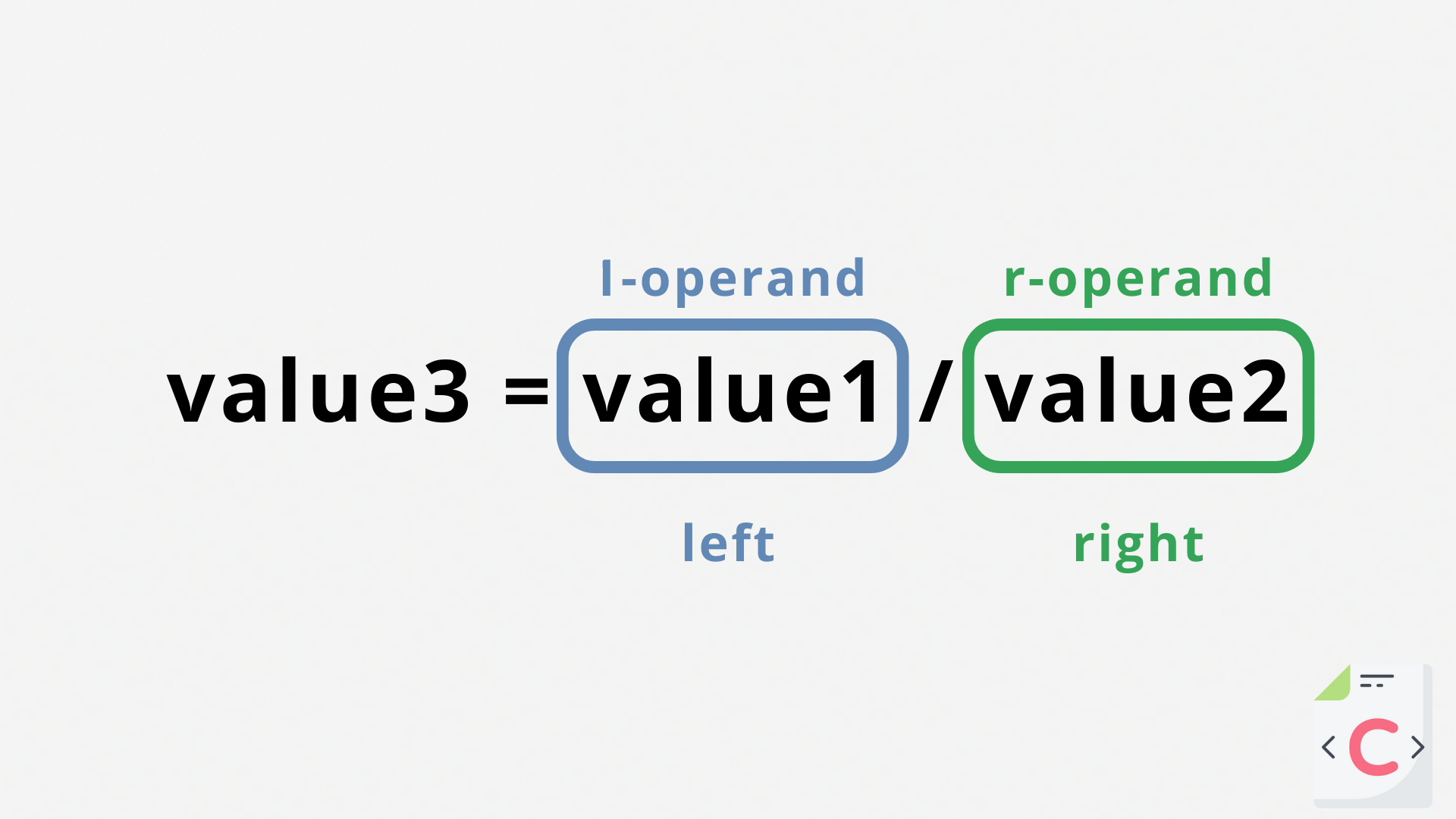Course Content

C Basics

##Multiplication, Division and Modulo

Multiplication (*)

The `*` multiplication operator forms the product of the values on both sides of the "*" sign.

For example, let's calculate how many liters of water we need to completely fill the pool:Main.cDivision (/)

The division operator `/` divides the right operand's value by the left operand's value.For example, let's find out the speed of the car:Main.cThe `double` variables were created to make our answer non-integer and, therefore, more accurate. Otherwise, if we manipulate only integer values, the result will also be an integer:Main.cMaybe it will work if you change the specifier? – No.

The result will be completely incorrect:Main.cBut there is also a correct calculation option without an additional variable:Main.cNote

To have a non-integer result, one of the variables must be non-integer.

Modulo (%)

Operator `%` returns the remainder of the normal division. For example:Main.cNote

We used the `%` character twice in a row to warn the compiler that we wanted to use the `%` character as a string, not as a specifier.